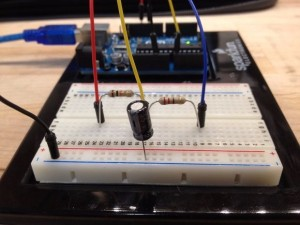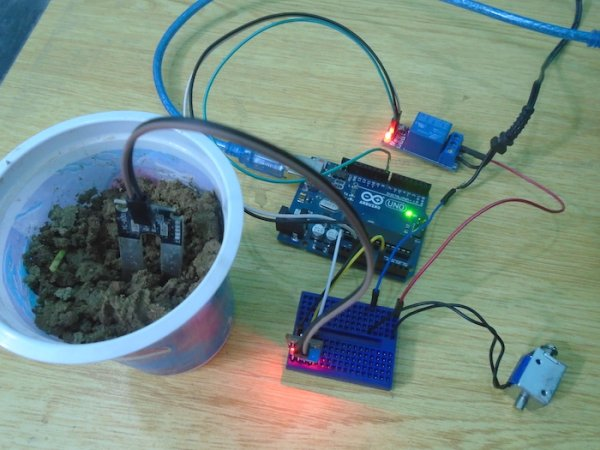Measure Capacitance with Arduino

This tutorial provides a guide on how to set up an Arduino to measure the capacitance of a capacitor. This can be useful if the capacitor is unlabeled or if it is self-built.Capacitance is an object’s ability to store an electric charge. Reasonably, this object is referred to as a capacitor. A capacitor that stores this charge in an electric field between two conductive plates is known as a parallel plate capacitor. The non-conductive material that is between these two plates is known as a dielectric. Dielectrics change the amount of charge a capacitor can hold and , in practice, what the particular capacitor would be used for (e.g. high frequency circuits, high voltage circuits, etc).

The equation for the capacitance of a parallel plate capacitor is:

For More Details: Measure Capacitance with Arduino

This Post / Project can also be found using search terms:

• how to measure cpacitance using arduino mega
• measure capacitance arduino
• using arduino to measure capacitance of parallel plate
READ  Missed the Maker Faire? Catch the pictures!